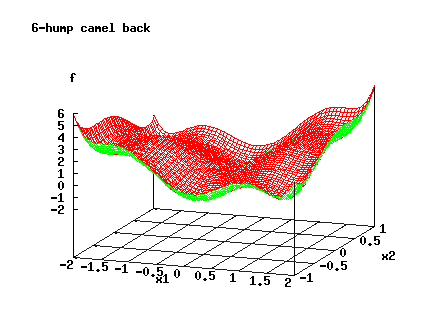Global Optimization

The occurrence of multiple extrema makes problem solving in nonlinear optimization even harder. Usually the user dreams of the global (best) minimizer, which might be difficult to obtain without supplying global information, which in turn is usually unavailable for a nontrivial case. The following picture shows the function

f(x,y)=x^2*(4-2.1*x^2+x^4/3)+x*y+y^2*(-4+4*y^2)

which has two global minima at (0.09,-0.71) and (-0.09,0.71) and four additional local minima.The Unconstrained NLO-Problem:

min f(x), n=dim(x).

Global optimization is a difficult area, at least for larger n, since there is no easy algebraic characterization of global optimality. Most of the codes designed for minimization simply restrict themself to solve the equation grad(f(x))=0, which is only necessary of course. Methods using interval-arithmetic and branch&bound will in principle solve these problems, but the branch tree might become excessively large for difficult functions. Hence for higher dimensions and without special structure only stochastic or pseudostochastic methods will apply.
See however the books of Kearfott Rigorous Global Search: Continuous Problems Kluwer, Dordrecht 1996, Floudas Deterministic Global Optimization: Theory, Algorithms and Applications , Kluwer, Dordrecht 1999, and Hansen&Walster Global Optimization Using Interval Analysis , Marcel Dekker 2003. See A. Neumaier's paper on methods and software as well as J. Pinter's survey on available software.

Mostly stochastic or heuristic search methods have been available freely, but this has changed, see below for constrained optimization.

 DFL A library of algorithms for derivative free (global) optimization (f90, C, Julia) PANMIN Two stochastic methods, one MPI parallelized; uses Merlin/MCL BTRK f90-version of the derivative-free Boender-Timmer-Rinnoy Kan algorithm PSwarm Hybrid derivative-free solver (particle swarm & direct search), AMPL interface (C (also parallel), Matlab) MEMPSODE Hybrid derivative-free solver (particle swarm & local search); uses Merlin/MCL MLOCPSOA particle swarm paradigm, AMPL interface (C source, binaries) netlib/toms/667 method of Zirilli et al (stochastic differential equation approach) GANSO several methods for global&nonsmooth problems (C/C++) netlib/opt/simann simulated annealing (f77) simannf90 f90-version from Alan Miller simann.m Matlab version Anneal C/C++/Ada/Forth implementations of SA Java-SA Java implementation of SA Simanneal Python implementation of SA CSA Python implementation of coupled SA ASA C, adaptive simulated annealing, Python interface CMA-ES Evolution Strategy with Covariance Matrix Adaptation (Matlab) PGSL A direct stochastic algorithm for global search incl constraints (C) Fortran-GA genetic algorithm PIKAIA genetic algorithm; IDL, f90, parallel versions Excel version DGPF Distributed Genetic Programming Framework. needs Eclipse for compile SIGOA Simple Interface for Global Optimization Algorithms, Java source

These methods are simple to use, but require a high number of function evaluations if you desire acceptable final precision. Here are codes which determine the global minimum of a Lipschitz continuous function on a finite box:

 DIRECT ( f77, Matlab, C, Julia) using a bound for the Lipschitz constant of f and subdivision MCS Huyer&Neumaier's Multilevel Coordinate search, local search by SQP, Matlab

The following codes fit a surrogate model to an expensive to evaluate function of few variables and analyze/minimize that:

 SPACE global optimization through meta-modeling: fitting, cross-validation, prediction, minimization (C++) Spacemap Optimization using Surrogate Models (Matlab)

The Constrained NLO-Problem:

min f(x) subject to h(x)=0, g(x)>=0,
n=dim(x), m=dim(g), p=dim(h).

Few codes are available but this is an area of current research and more links will be added. See also the book by Eldon Hansen, Global Optimization Using Interval Analysis, Dekker, New York, 1992.

For a good introduction into the theory see the book by Horst et al. For the state of the art until 1994, see the Horst&Pardalos handbook. An application-oriented overview is given in this book by Floudas. An introduction is given by Liberti and recent survey papers on the mixed-integer case are Burer et al and Belotti et al

 Black Box Opt Black box functions and constraints, expensive evaluations, no derivatives needed (C, GPL) GloptiPoly Polynomial objective and constraints, integer variables, needs SeDuMi (Matlab, GPL) SOSTOOLS Matlab toolbox to solve sum of squares polynomial problems; needs SeDuMi and Matlab's symbolic toolbox (GPL) INTLAB Matlab toolbox for self-validating algorithms DIRDFN A DiRect algorithm with derivative-free local searches for general constrained global optimization problems (Fortran90) DIRECT version of DIRECT that handles general constraints using L1 penalty (Matlab) GlobSol Interval software, f90, book, exhaustive search, automatic differentiation, bound and equality constraints, nonlinear systems, sources, needs several local optimizers and linear algebra routines plus the author's interval package VerGO C++, interval branch&bound, bound constraints, automatic differentiation, self-contained Genocop various versions, including for nonlinear constraints (C) PGSL A direct stochastic algorithm for global search incl constraints (C) BARON solves variety of global problems deterministically including discrete, free demo version from GAMS COUENNE solves continuous and discrete global problems deterministically, C++ SCIP solves continuous and discrete global problems deterministically, C Maple GOT toolbox containing both B&B and random methods